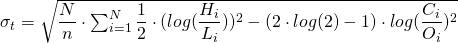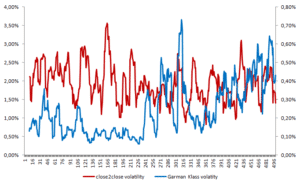# Garman Klass volatility

The Parkinson volatility estimate adjusts the regular volatility calculation by using the high and low prices of the day to estimate the variability. Although this is a valuable extension, it does not take into account the opening and closing price. Since markets are most active during the opening and closing of a trading session, this is an non-negligible shortcoming. The Garman Klass volatility is a type of volatility estimator that incorporates some intraday information stored at waily frequencies.

## Garman Klass volatility formula

The Garman Klass volatility estimator is calculated as follows. First start by determining the scaling factor. This equals to N, the amount of trading days in a year, and n, your chosen sample size. Note that when n equals the amount of trading days in a year, there is no scaling required. Thereafter, this scaling factor is multiplied with the variance of the sample (the sum in the equation). As such, we get the annualized Garman Klass variance. By taking the square root over this result, we get the Garman Klass volatility estimator.## Garman Klass volatility benefits and drawbacks

One obvious benefit is that this volatility estimator incorporates intraday variability information to estimate the volatility. Just like in Parkinson volatility estimator, this is of high relevance for investor that take leverage. These investors should properly take care of meeting intraday margin requirements when implementing their trading strategies. A drawback of this measure, is that volatility is not robust for opening jumps in prices. Overnight jumps bias the estimate and is thus not ideal for use.## Summary

The Garman Klass volatility estimtor incorporates the some intraday information on price variability. More specifically, the open, low, high and close price of a security.

### Garman Klass volatility

Want to have an implementation in Excel? Download the Excel file: Garman Klass volatility